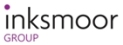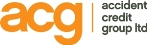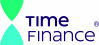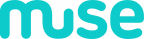# Mathematical Factoring Resources News

We have had a number of web viewers looking for information and help with mathematical factoring, the division of numbers into their factors. Therefore we have added a page with links to mathematical factoring resources and calculators: Mathematical Factoring

Posted: 20th of September 2014

Examples of funders we work with: